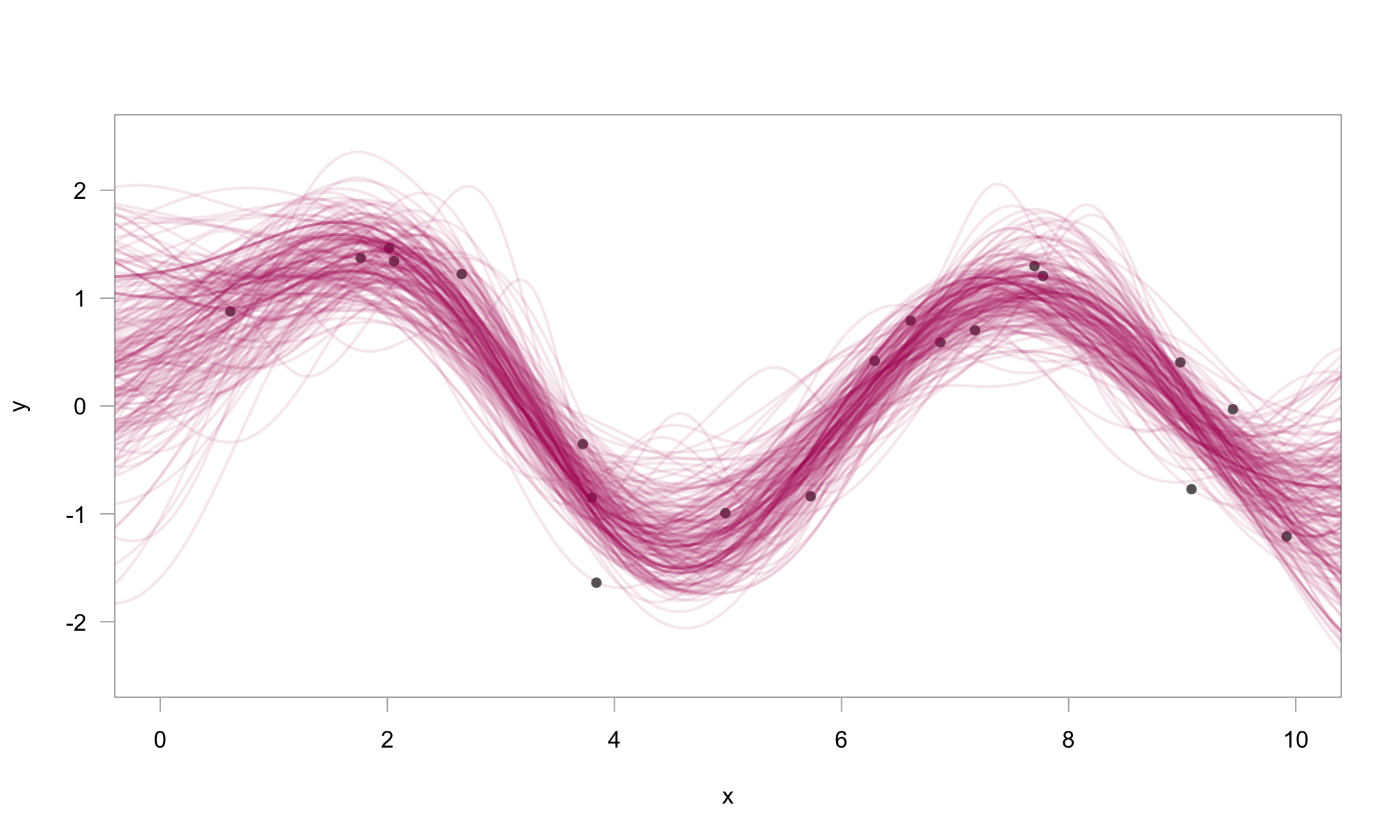greta.gp extends greta to let you define Gaussian processes as part of your model. It provides a syntax to create and combine GP kernels, and use them to define either full rank or sparse Gaussian processes.

## Installation

You can install the current release version of greta.gp from CRAN:

install.packages("greta.gp")

Or install the development version of greta.dynamics from r-universe:

install.packages("greta.gp", repos = "https://greta-dev.r-universe.dev")

You can also install the development version of greta.gp via GitHub:

remotes::install_github("greta-dev/greta.gp")

## Example usage

# simulate data
x <- runif(20, 0, 10)
y <- sin(x) + rnorm(20, 0, 0.5)
x_plot <- seq(-1, 11, length.out = 200)
library(greta)
library(greta.gp)

# hyperparameters
rbf_var <- lognormal(0, 1)
rbf_len <- lognormal(0, 1)
obs_sd <- lognormal(0, 1)

# kernel & GP
kernel <- rbf(rbf_len, rbf_var) + bias(1)
f <- gp(x, kernel)

# likelihood
distribution(y) <- normal(f, obs_sd)

# prediction
f_plot <- project(f, x_plot)
# fit the model by Hamiltonian Monte Carlo
m <- model(f_plot)
draws <- mcmc(m, n_samples = 250)
# plot 200 posterior samples
# plot 200 posterior samples
plot(
y ~ x,
pch = 16,
col = grey(0.4),
xlim = c(0, 10),
ylim = c(-2.5, 2.5),
las = 1,
fg = grey(0.7),
)
for (i in 1:200) {
lines(draws[][i, ] ~ x_plot,
lwd = 2,
col = rgb(0.7, 0.1, 0.4, 0.1))
}## Code of Conduct

Please note that the greta.gp project is released with a Contributor Code of Conduct. By contributing to this project, you agree to abide by its terms.